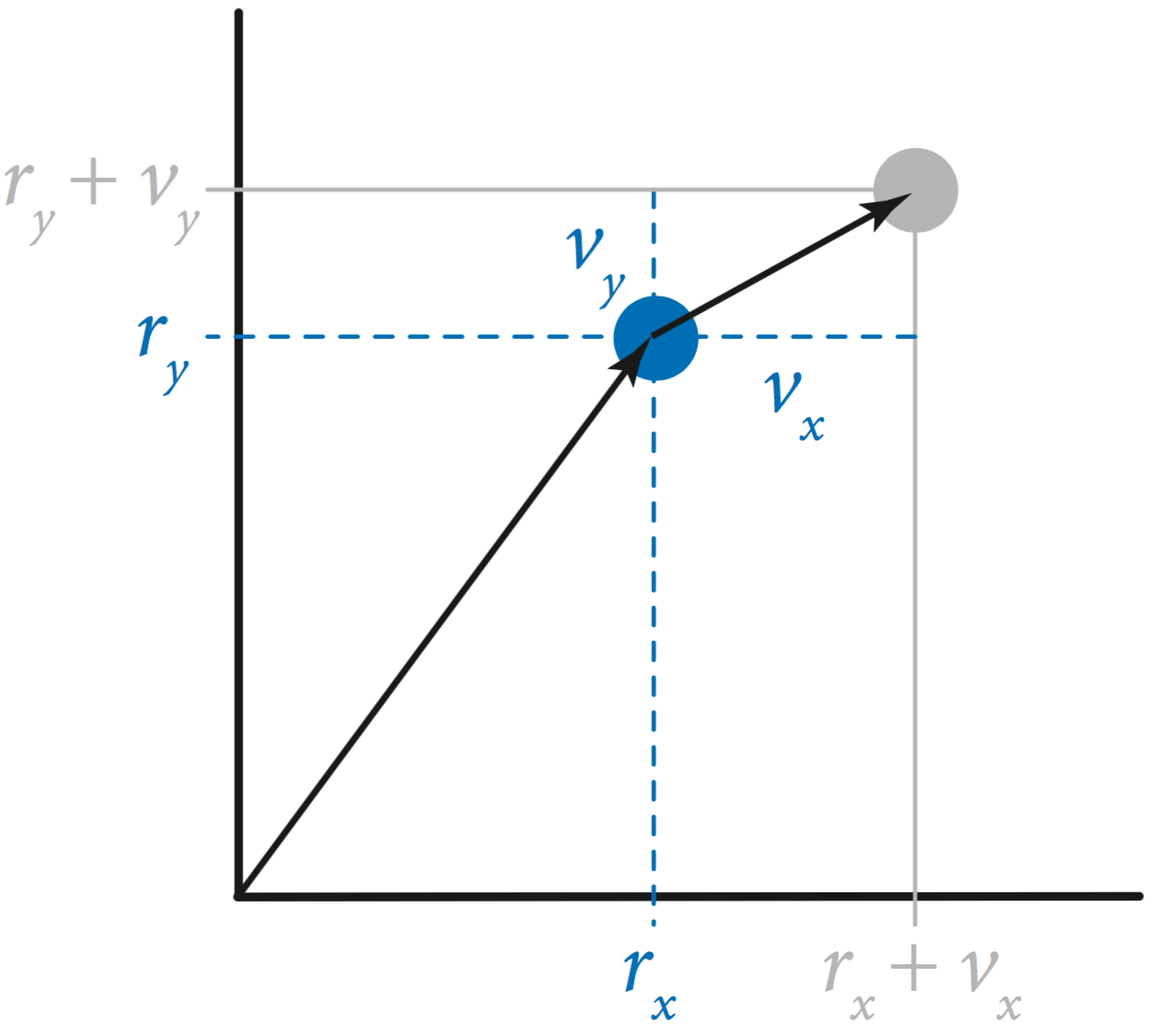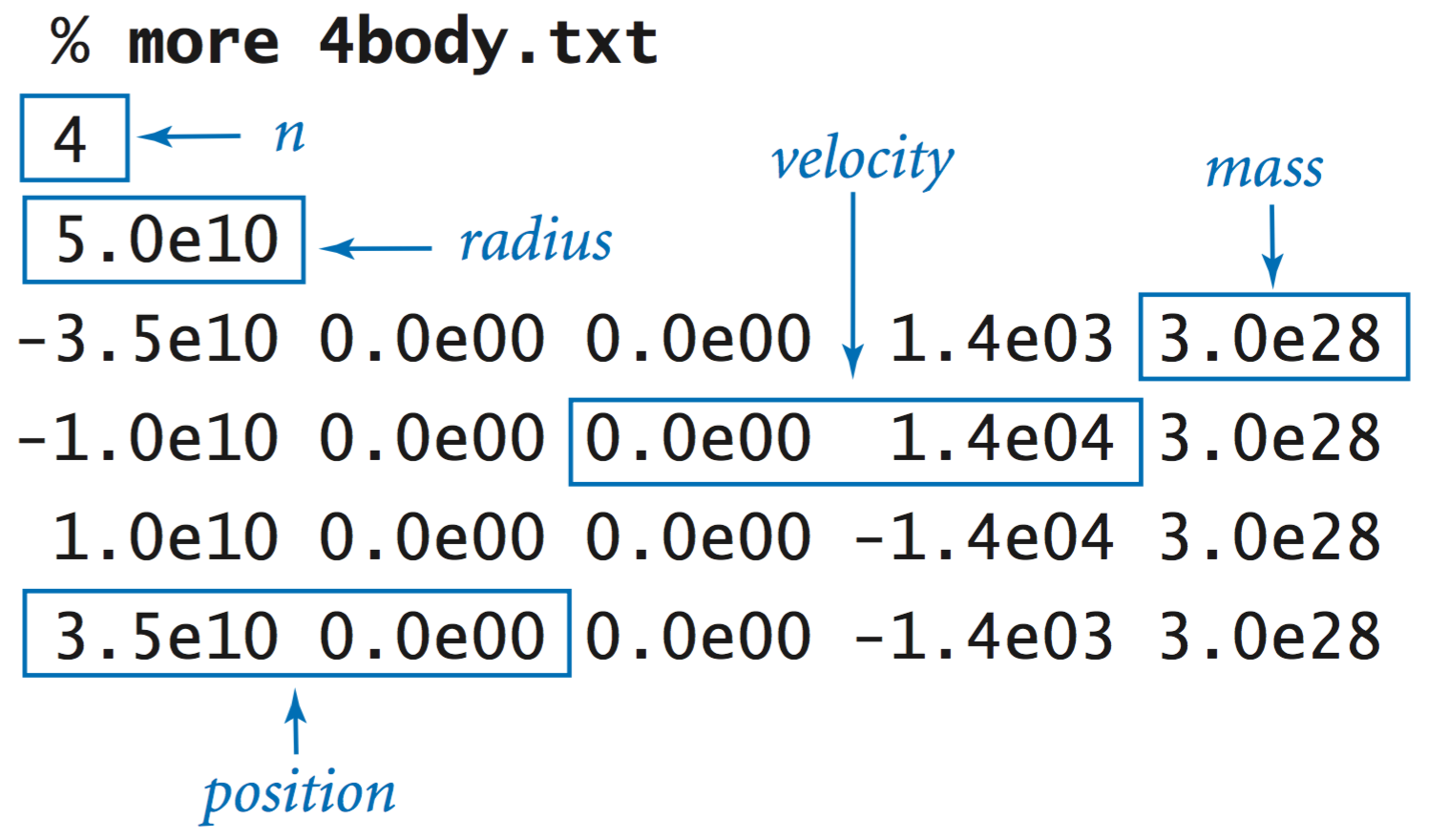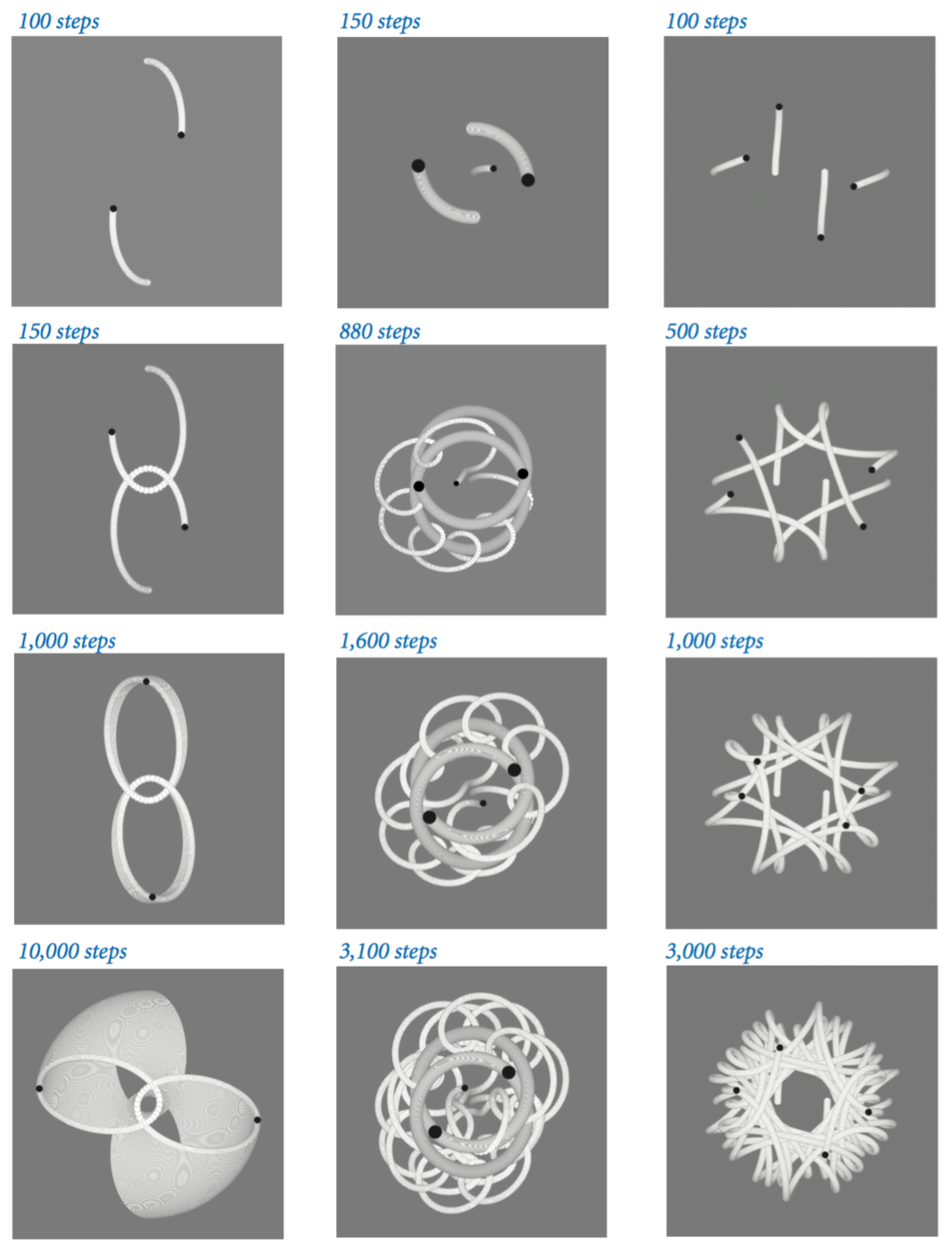# 3.4   Case Study:   N-body Simulation

In this section, we write an object-oriented program that dynamically simulates the motion of n bodies under the influence of mutual gravitational attraction.

## Bouncing balls.

The data type Ball.java represents a ball with a given position $$(r_x, r_y)$$ that moves with a fixed velocity $$(v_x, v_y)$$ in the box with coordinates between −1 and +1. When it collides with the boundary, it bounces according to the law of elastic collision.The client BouncingBalls.java takes a command-line argument n and creates n random bouncing balls.

## N-body simulation.

The bouncing ball simulation is based on Newton's first law of motion: a body in motion remains in motion at the same velocity unless acted on by an outside force. Embellishing that example to incorporate gravity leads us to a basic problem that has fascinated scientists for ages. Given a system of n bodies, mutually affected by gravitational forces, the problem is to describe their motion.
• Body data type. The data type Body.java represents a body with a given position $$(r_x, r_y)$$, velocity $$(v_x, v_y)$$, and mass $$m$$. It applies Newton's third law of motion (which explains the gravitational force between two bodies) to determine the net force acting on a body:
$$\boldsymbol{F} = G \frac{m_1 m_2}{r^2}$$
and Newton's second law of motion (which explains how outside forces directly affect acceleration and velocity).
$$\boldsymbol{F} = m \boldsymbol{a}$$
It uses the Vector.java data type to represent displacement, velocity, and force as vector quantities.• Universe data type. Universe.java takes a command-line argument dt, reads in a universe from standard input, and simulates the universe using time quantum dt. Here is an example of the data file format:The following static images for 2body.txt, 3body.txt, and 4body.txt are made by modifying Universe.java and Body.java to draw the bodies in white, and then black on a gray background.#### Exercises

1. Develop an object-oriented version of BouncingBall.java from Section 1.5. Include a constructor for that starts each ball moving in a random direction at a random velocity (within reasonable limits) and a test client that takes an integer n from the command line and simulates the motion of n bouncing balls.

Solution: Ball.java and BouncingBalls.java.

2. What happens in a universe where Newton's second law does not apply? This situation would correspond to forceTo() in Body always returning the zero vector.

Solution: The bodies will move in straight lines, according to their initial velocities.

#### Creative Exercises

1. N-body simulation trace. Write a client UniverseTrace.java that produces traces of the n-body simulation system like the static images in the book.

#### Web Exercises

1. Colored bouncing balls. Modify Ball.java and BouncingBalls.java to associate a color with each ball. Name your programs ColoredBall.java and BouncingColoredBalls.java.
2. Friction and drag. Modify Ball.java to incorporate friction and drag. Name your data type DeluxeBall.java.
3. Generative music based on a gravity simulator. Generate music based on an n-body simulation where bodies makes notes when they collide. Simran Gleason's website describes the process and includes example videos.